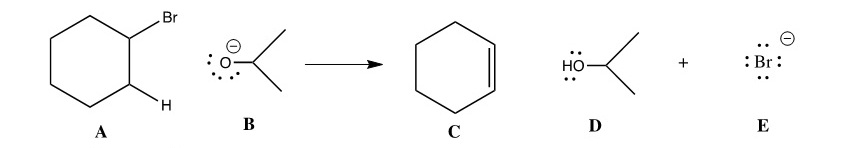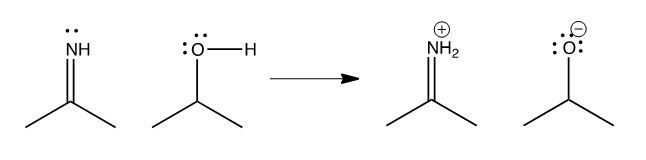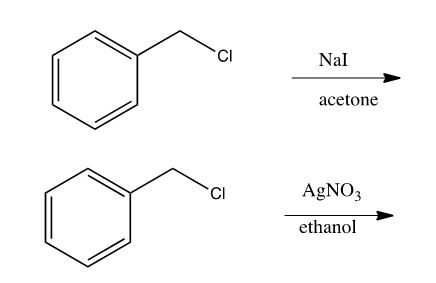Exam questions are a primary medium by which students learn what their instructor values most in the course. If we evaluate what we value, questions should test the mechanism and thus emphasize conceptual understanding, utilize real applications, and require deep thinking. And for me, the most important reason to pose mechanistic questions is to see how much students really know and understand.

Questions need not be completely different from more traditional exam questions but they must uncover why and how a reaction occurs, rather than just what occurs. When writing assessment questions for my course, ideas come from a variety of places. I often use the format of a question from Joel’s book or from Smartwork problems assigned for homework, while changing the context enough so that students can demonstrate that they understand the principles of the problem. I use questions students ask during office hours or study sessions as seeds for new assessment questions; if one student’s learning hinges on a given concept then others can probably learn from the question, too. Some exam questions I use are derived from clicker questions for which the majority of the class responded incorrectly, as I use these questions as opportunities to address misconceptions in class. The resulting exam questions allow me to measure student growth.

Here are some examples of questions I would have asked under a traditionally organized course and how they evolved for my mechanistically organized course:

Question 1
Original version:

In the following reaction,                              is the nucleophile, and __________ is the electrophile.Testing the mechanism version using the same figure:

For the following reaction, identify all electron-rich and electron-poor sites in the starting materials, and supply the missing mechanism arrows required to form the given product.

The original version of the question was used after the substitution and elimination reaction chapter were complete, and tested the students’ recognition of the labels used for the reaction. Students could answer successfully by remembering that many nucleophiles have negative charges or that alkyl halides are often electrophiles. The new question delves into the reason for the nucleophilic and electrophilic character. This question was asked right after the Ten Elementary Steps chapter, before students had studied any particular reaction mechanism, demonstrating the generality of the concepts. Despite the early point in the course, over 80% of my students earned at least partial credit on this question.

Question 2
Original version:

Consider the acid/base reaction below:(a) Label and acid, base, conjugate acid, conjugate base in this reaction.
(b) Indicate which side would predominate at equilibrium.

Testing the mechanism question using the same figure:

(a) Draw curved arrows to describe acid/base reaction going from left to right.
(b) Clearly indicate which acid/base combination is predominant at equilibrium (on which side will the equilibrium lie?).
(c) Draw an energy diagram for this process, with reaction coordinate (left to right) on the x-axis, and energy on the y-axis.

The old question required students to recognize proton donors/acceptors and to pick from a list of criteria for what makes a stronger acid. The new question replaced the labeling of acids and bases with the curved arrows showing electron flow for proton transfer and asked students to equate preferred species at equilibrium with energy, expressed on an energy graph. Most students in my class attempted the revised question with reasonable success on parts (a) and (b). Part (c) proved daunting for many students, and only about half attempted it. Of these students, almost all drew graphs showing a DH consistent with the answer to part (b), but only a few added a transition state for a single step reaction.

Question 3
Original version:

Indicate the principle pathway by which each of the two reactions below would proceed (SN1, SN2, E1, E2):Testing the Mechanism question using the same figure:

Benzyl chloride reacted rapidly in both the SN2 and SN1 reactions in the laboratory. Show the rate determining step (curved arrows and product of the step) for each of these reactions, and explain why both reactions occurred rapidly, using words and resonance structures as appropriate.

To answer the original questions, students would have to recall that primary benzylic compounds could undergo either unimolecular or bimolecular substitution (but not elimination) and use nucleophile strength and/or solvent properties to choose the correct pathway. The revised question requires students to use the mechanism for the rate determining step to explain the observed reaction rates. A complete answer allows students to demonstrate an understanding of both steric and electronic effects of the substrate on reaction outcome. Upon grading the question I learned that most of my students did not understand that I wanted them to explain how the structure of benzyl chloride supported rapid reaction under two different mechanisms. Most did, however, provide a valid mechanism for the rate determining step of each reaction! While I’m learning to test the mechanism effectively, I’m also reminded that careful structuring of questions is needed to elicit responses I expect. In the future I would revise this question further, as follows:

Benzyl chloride reacted rapidly in both the SN2 and SN1 reactions in the laboratory. Show the rate determining step (curved arrows and product of the step) for each of the reactions below. Then, separately explain how the same substrate, benzyl chloride can react rapidly using two different mechanism. Use words and draw resonance structures for your response as appropriate.

Assessments, whether formative classwork/homework or summative exam questions, communicate to students what we as instructors value. I believe that careful preparation of evaluation questions can greatly influence what and how our students learn, and constructing and choosing appropriate evaluation is one of the most important things we do. Evaluating the mechanism allows instructors to learn just what their students are thinking. Proper evaluation is the antithesis of teaching to the test, and is not just testing what we teach. I like to think of thoughtful assessment as evaluating the learning we value, and I value the students’ deep learning through mechanism.

-Kimberley Cousins, California State University-San Bernardino